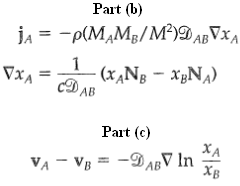### Create an Account

Home / Questions / Equivalence of various forms of Fick's law for binary mixtures a Starting with Eq A of Tab...

# Equivalence of various forms of Fick's law for binary mixtures a Starting with Eq A of Table 17.8-2 derive Eqs Starting with Eq A of Table 17 8 2 derive the folowing

Equivalence of various forms of Fick's law for binary mixtures
(a) Starting with Eq. (A) of Table 17.8-2, derive Eqs. (B), (D), and (F).
(b) Starting with Eq. (A) of Table 17.8-2, derive the folowing flux expressions: what conclusions can be drawn from these two equations?
(c) Show that Eq. (F) of Table 17.8-2 can be writtenasJun 12 2020 View more View LessSubscribe To Get Solution